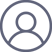诸平AI将一个10万个方程的量子物理问题简化为只有4个方程精选
2022-9-29 14:47

AI将一个10万个方程的量子物理问题简化为只有4个方程A visualization of a mathematical apparatus used to capture the physics and behavior of electrons moving on a lattice. Each pixel represents a single interaction between two electrons. Until now, accurately capturing the system required around 100,000 equations—one for each pixel. Using machine learning, scientists reduced the problem to just four equations. That means a similar visualization for the compressed version would need just four pixels. Credit: Domenico Di Sante/Flatiron Institute

“我们从所有这些耦合在一起的微分方程的巨大对象开始;然后我们用机器学习（machine learning）把它变成小到可以用手指数的东西，”该研究的主要作者、纽约熨斗研究所计算量子物理中心{Flatiron Institute's Center for Computational Quantum Physics (CCQ) in New York City}的访问研究员、意大利博洛尼亚大学的助理教授多梅尼科·迪桑特（Domenico Di Sante）说。

Neural networks and 'ghost' electrons accurately reconstruct behavior of quantum systems

Abstract

We perform a data-driven dimensionality reduction of the scale-dependent four-point vertex function characterizing the functional renormalization group (FRG) flow for the widely studied two-dimensional tt′ Hubbard model on the square lattice. We demonstrate that a deep learning architecture based on a neural ordinary differential equation solver in a low-dimensional latent space efficiently learns the FRG dynamics that delineates the various magnetic and d-wave superconducting regimes of the Hubbard model. We further present a dynamic mode decomposition analysis that confirms that a small number of modes are indeed sufficient to capture the FRG dynamics. Our Letter demonstrates the possibility of using artificial intelligence to extract compact representations of the four-point vertex functions for correlated electrons, a goal of utmost importance for the success of cutting-edge quantum field theoretical methods for tackling the many-electron problem.